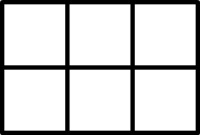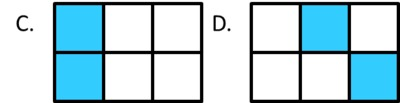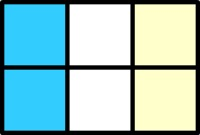Halves, thirds, and sixths

Alignments to Content Standards: 3.MD.C.6 3.G.A.2 3.NF.A.1 3.NF.A.3.b

1. A small square is a square unit. What is the area of this rectangle? Explain.2. What fraction of the area of each rectangle is shaded blue? Name the fraction in as many ways as you can. Explain your answers.3. Shade $\frac12$ of the area of rectangle in a way that is different from the rectangles above.4. Shade $\frac23$ of the area of the rectangle in a way that is different from the rectangles above.IM Commentary

The purpose of this task is for students to use their understanding of area as the number of square units that covers a region (3.MD.6), to recognize different ways of representing fractions with area (3.G.2), and to understand why fractions are equivalent in special cases (3.NF.3.b). Determining the fraction of the area that is shaded for rectangles A-D in part (b) is increasingly complex. Rectangles E, F, and G show that there are many ways for $\frac12$ of the area to be shaded blue, which implies that there are many ways to represent the fraction $\frac12$ with area. Rectangle H requires students to see the equivalence of two fractions, neither of which is a unit fraction. Students get a chance to demonstrate what they have learned in part (b) by generating their own representations of fractions in parts (c) and (d).

Note that in third grade, students are limited to working with halves, thirds, fourths, sixths, and eighths. While it would be acceptable in instructional situations to work with other fractions, students should have an opportunity to work extensively with the fractions mentioned in order to develop a deep and flexible understanding of them. In particular, summative assessment should be strictly limited to fractions with denominators 2, 3, 4, 6, and 8.

This is an instructional task. Students would benefit from having tracing paper, colored pencils, and multiple blank copies of the rectangle as they work. Students would also benefit from working in pairs or small groups so they can compare their answers and explain the fraction equivalences to each other.

Attached Resources

• Lesson Plan - Unit Fractions 1
• Solution

1. The area of the the rectangle is 6 square units.
• $\frac16$ of rectangle A is shaded blue because the area is 6 square units and one square unit is shaded.
• $\frac36$ of rectangle B is shaded blue because the area is 6 square units and 3 square units are shaded. We can also say that $\frac12$ of rectangle B is shaded blue because half of the squares are shaded. This shows that $\frac36$ and $\frac12$ are equivalent fractions.
• $\frac26$ of rectangle C is shaded blue because the area is 6 square units and two square units are shaded. We can also say that $\frac13$ of rectangle C is shaded blue. This is easier to see if we add some more shading:We can see that the part that is shaded blue is the same size and shape as the part that is shaded yellow and the part that is white, so each of these parts is $\frac13$ of the rectangle. This shows that $\frac26$ and $\frac13$ are equivalent fractions.
• $\frac26$ of rectangle D is shaded blue because the area is 6 square units and two square units are shaded. We can also say that $\frac13$ of rectangle D is shaded blue. We can cut figures apart and rearrange them without changing the area (as long as the pieces don't overlap when we are done). We can see that $\frac13$ of the area is shaded if we rearrange the squares so it looks like rectangle C.
• $\frac36$ of rectangle E is shaded blue because the area is 6 square units and 3 square units are shaded. We can also say that $\frac12$ of rectangle E is shaded blue because half of the squares are shaded. We can also rearrange them to look like rectangle B. This shows that $\frac36$ and $\frac12$ are equivalent fractions.
• $\frac36$ of rectangle F is shaded blue because the area is 6 square units and 3 square units are shaded. We can also say that $\frac12$ of rectangle F is shaded blue because half of the squares are shaded. We can also see that the three blue squares form a "piece" of the rectangle that is the same size and shape as the piece formed by the three white squares. This shows that $\frac36$ and $\frac12$ are equivalent fractions.
• $\frac36$ of rectangle G is shaded there are 6 equal squares and three are shaded. We can also say that $\frac12$ of rectangle G is shaded blue because half of the squares are shaded. We can also rearrange them to look like rectangle B. This shows that $\frac36$ and $\frac12$ are equivalent fractions.
• $\frac46$ of rectangle H is shaded there are 6 equal squares and three are shaded. We can also say that $\frac23$ of rectangle H is shaded blue because as we saw earlier, two blue squares represent $\frac13$ of the rectangle and two "2-square rectangles" are shaded. This shows that $\frac46$ and $\frac23$ are equivalent fractions.
2. There are many ways to do this.
3. There are many ways to do this.